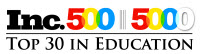8th Grade MathematicsCourse Number:   CMN2877Course Overview
Format What's This?
Structure What's This?
InstitutionFOUNDERS Education
SubjectMathematics Core
Education LevelMiddle School
Course Fee
Registration

Help
Personal Learning CoachTMTo Be Assigned
PLC Emailplc@educere.net
PLC Toll-Free Number866.4EDUCERE (866.433.8237)
* Prospective NCAA Division I and II student-athletes should visit the NCAA Eligibility Center website (www.eligibilitycenter.org) and seek the NCAA's acceptance of a particular Education Provider's virtual course prior to registration.

Course Description
In this course, students use linear equations and systems of linear equations to represent, analyze, and solve a variety of problems. They understand that the slope of a line is a constant rate of change, so that if the input or x-coordinate changes by an amount A, the output or y-coordinate changes by the amount mÃ‚Â·A. Students also use a linear equation to describe the association between two quantities in bivariate data (such as arm span vs. height for students in a classroom). Students use linear equations, systems of linear equations, linear functions, and their understanding of slope of a line to analyze situations and solve problems. Students grasp the concept of a function as a rule that assigns to each input exactly one output. They can translate among representations and partial representations of functions (noting that tabular and graphical representations may be partial representations), and they describe how aspects of the function are reflected in the different representations. Students use ideas about distance and angles, how they behave under translations, rotations, reflections, and dilations, and ideas about congruence and similarity to describe and analyze two dimensional figures and to solve problems. Students apply the Pythagorean Theorem to find distances between points on the coordinate plane, to find lengths, and to analyze polygons. Students complete their work on volume by solving problems involving cones, cylinders, and spheres.

Delivery Method
• Internet
• Real-Time Web-Based Text Meeting Access

Courses and course detail are subject to change without notice.
 educere@educere.net toll-free 866.4EDUCERE (866.433.8237) telephone 215.283.0380 fax 866.348.1050Educere LLC Ambler Plaza 12 East Butler Avenue, Suite 100 Ambler, PA 19002